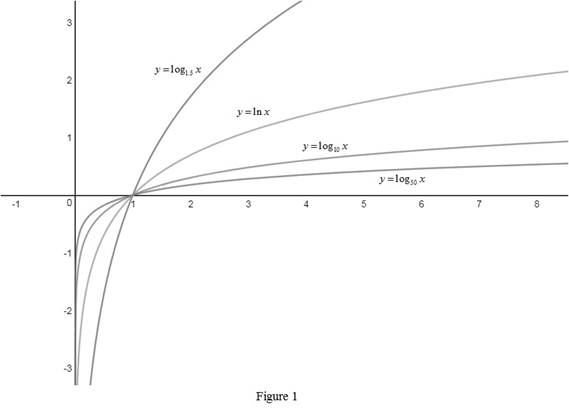# Use Formula 10 to graph the functions y = log 1.5 x , y = ln x , y = log 10 x and y = log 50 x on a common screen and interpret how these graphs is related.### Single Variable Calculus: Concepts...

4th Edition
James Stewart
Publisher: Cengage Learning
ISBN: 9781337687805### Single Variable Calculus: Concepts...

4th Edition
James Stewart
Publisher: Cengage Learning
ISBN: 9781337687805

#### Solutions

Chapter 1.6, Problem 43E
To determine

## To sketch: Use Formula 10 to graph the functions y=log1.5x,y=lnx,y=log10x and y=log50x on a common screen and interpret how these graphs is related.

Expert Solution

### Explanation of Solution

The graphs of the function y=log1.5x,y=lnx,y=log10x and y=log50x are drawn and shown below in Figure 1.From Figure 1, it is noted that the graphs are passing through the common point (1, 0).

Use Formula 10, logba=lnalnb,b1 and express the given log function in terms of natural logarithm (ln) and identify the relationship between each graph.

log1.5x=lnxln1.5log10x=lnxln10log50x=lnxln50

Thus, the given graphs are related in a such a way that y=lnx times the factor, y=1ln(base) .

### Have a homework question?

Subscribe to bartleby learn! Ask subject matter experts 30 homework questions each month. Plus, you’ll have access to millions of step-by-step textbook answers!